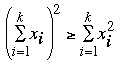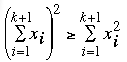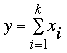SEARCH HOMEMath Central Quandaries & QueriesQuestion from Bhavya, a student: Prove by induction that if Xi >= 0 for all i, then (Summation Xi from 1 to n)^2 >= Summation Xi^2 from 1 to nHi,

First I suggest that you look at Victoria's response in The Principle of Mathematical Induction.

For her step 1. check that your statement is true for i = 1.

Step 2. assume that your statement is true for some n = k ≥ 1. Thus for your statement that is assume that for some k ≥ 1Step 3. is the inductive step. Based on the assumption in step 2. you are to prove that(*).

To prove this letthen the left side is (xi + y)2. Expand this using the binomial theorem and use the assumption in part 2. to conclude that statement (*) is true.

Perform step 4.

I hope thus helps,
PennyMath Central is supported by the University of Regina and The Pacific Institute for the Mathematical Sciences.